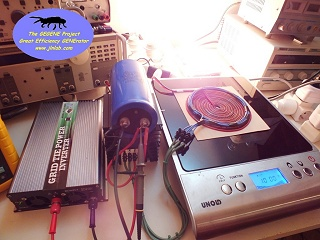TEST #3 : Efficiency measurement with a calorimetric method
created on 28 décembre, 2012 - JLN Labs - last update 2 Janvier 2013
All informations and diagrams are published freely (freeware) and are intended for a private use and a non commercial use.
Toutes les informations et schémas sont publiés gratuitement ( freeware ) et sont destinés à un usage personnel et non commercial

Cliquez ici pour la version FRANCAISE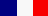January 2, 2013 - TEST #3 : Here is the result of the 3rd serie of measurements using a calorimetric method. For measuring the electrical power at the output of the flat bifilar coil, I have connected an electrical kettle and I have applied a calorimetric conversion method. I have used a 2400 W electric kettle (1.7 liter) from Moulinex "subito" model ref BY530D30. The kettle is filled with 1000 mL of water and its temperature is measured with an immersed thermal probe connected to an electronic thermometer HFT81 Bioblock. The duration of the heating is measured with a chronometer. The electrical power input for the induction cooker is measured with a Wattmeter Energy Logger 4000F directly connected on the power grid.

Below the TEST #3 setup :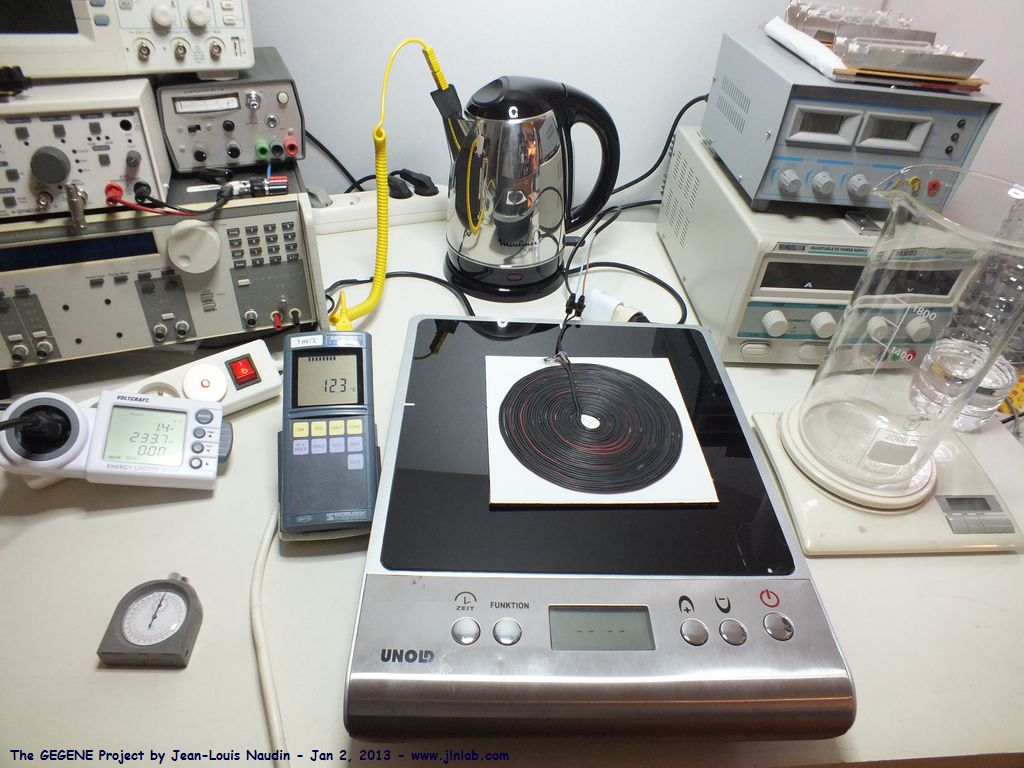The tools required for the TEST #3 are : a grid Wattmeter, an electronic thermometer, a chronometer and a calculator...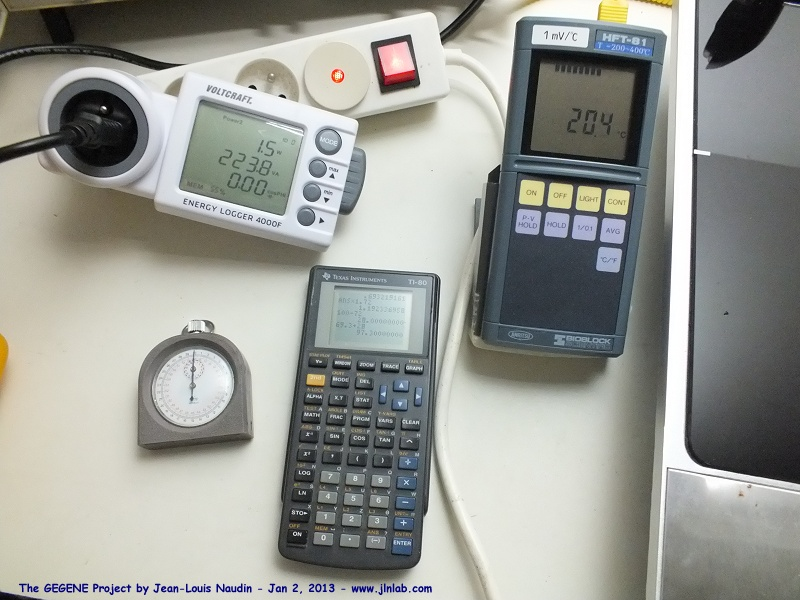and of course an electric kettle...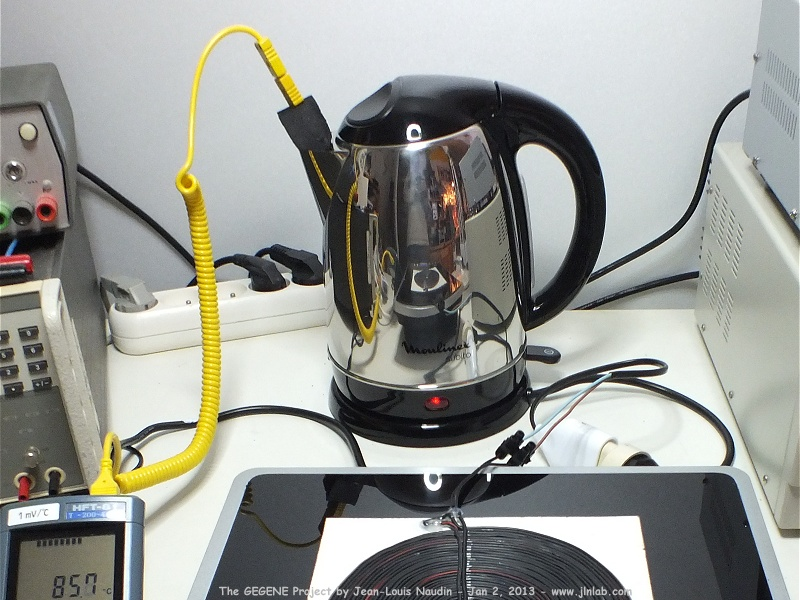1 - Calibration : Efficiency measurement of the electric kettle

Before proceeding to the measurement of the power at the output of the flat bifilar coil, a calibration phase is required. The measurement of the efficiency of the electric kettle is required. So, I have simply connected the kettle directly to the power grid via the Wattmeter. The kettle is filled with 1000 g of water (1000 mL).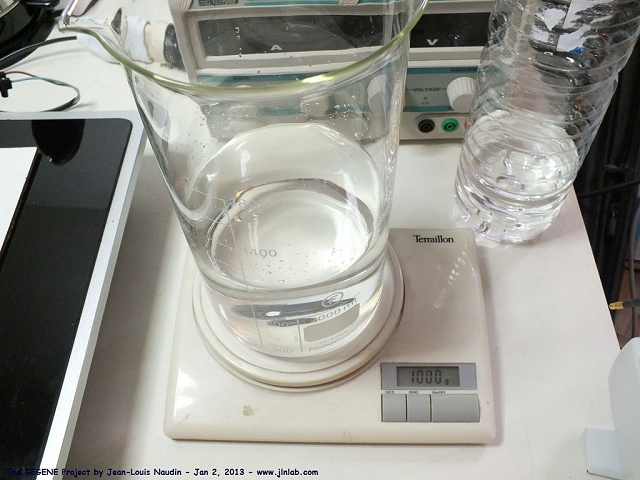The kettle is filled with 1000 mL of water and the thermal probe is immersed in the liquid.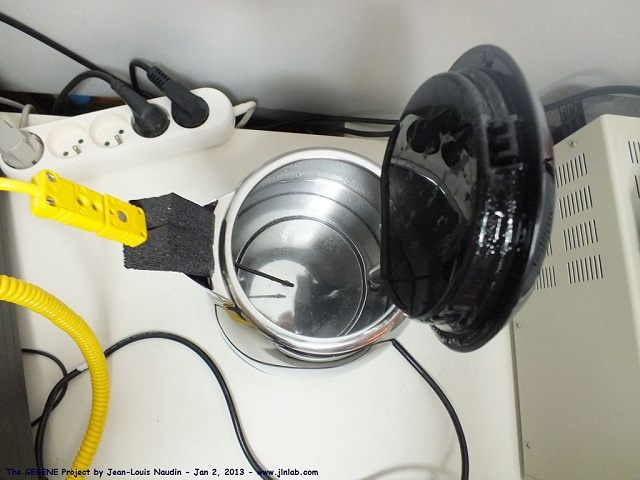The method is simple : We need 4180 Joules of energy to heat 1000 g of water up to 1°C. So, it is easy to calculate the energy required to heat up the water and thus to compute the efficiency knowing the electrical energy required to power the kettle. For instance, the energy efficiency to heat up 1000 mL of water from 20°C to 100°C is 80 times 4180 Joules.

Below, the calibration phase :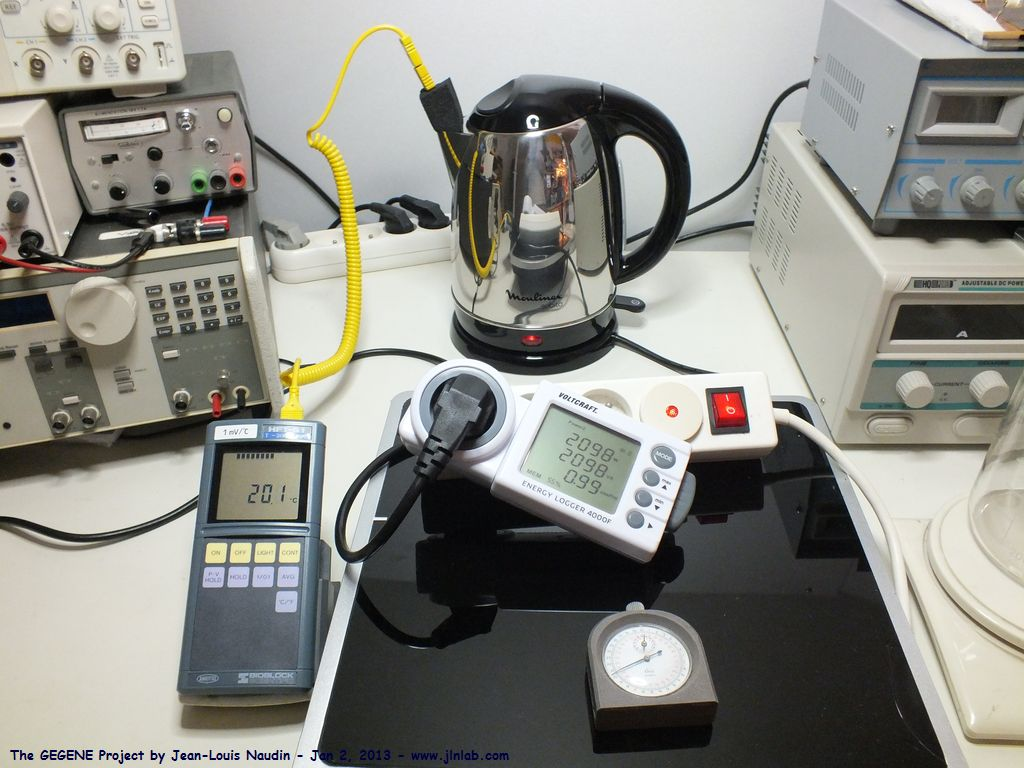Below the efficiency measurement of the electric kettle :

 Amount of water = 1000 g or 1000 mL Initial temperature Ti = 14.5°C Final temperature Tf = 96.6°C DT = Tf - Ti = 82.1 °C Heating duration t = 227 secondes Electrical Power at the input = 2100 Watts Energy required to heat the water : Wtherm = 4180 * 82.1 = 343178 Joules Input electrical energy : Welec = 2100 * 227 = 476700 Joules Efficiency of the kettle = Wtherm / Welec = 343178 / 476700 = 0.7199 the efficiency of the electrical kettle r = 72 % The correction factor, due to the loss in the kettle, to be applied is : 1/r = 1.38908

2 - Efficiency measurement of the GEGENE with the calorimetric method

Now that we have the thermal correction factor, the electrical kettle is connected to the output of the flat bifilar coil and the electrical power of the induction cooker is measured with the Wattmeter connected on the power grid.The kettle is filled again as previously with 1000 mL of cold water and the induction plate is programmed to provide 1000 W.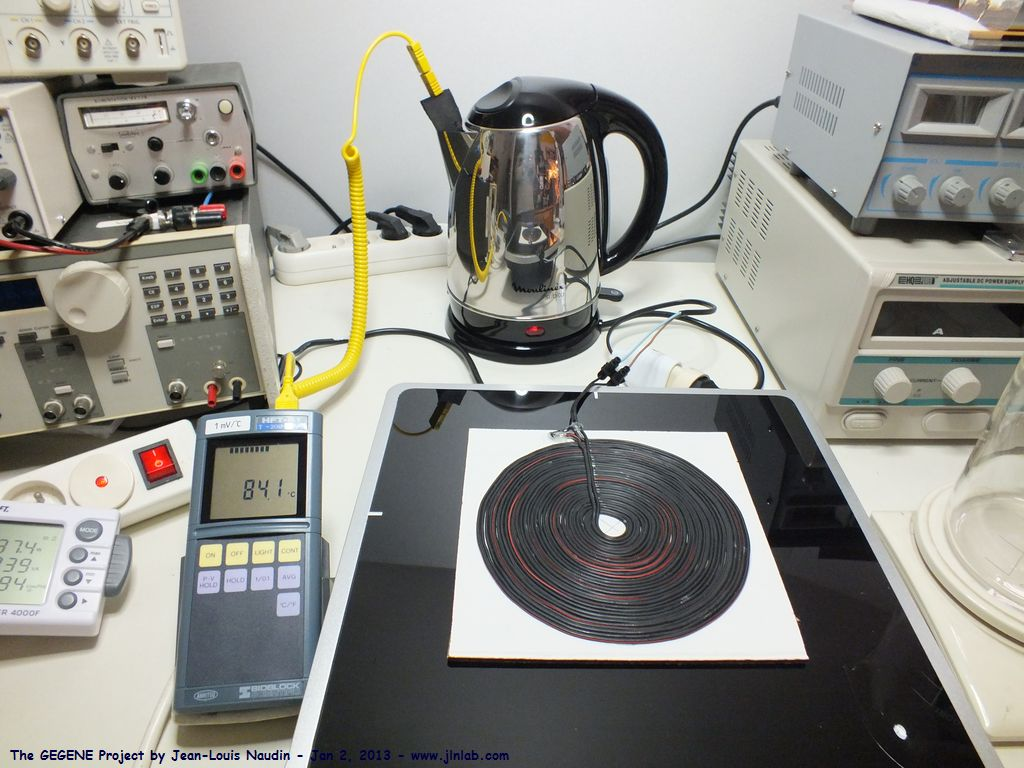Here the efficiency measurements results :

 Amount of water = 1000 g or 1000 mL Initial temperature Ti = 12.7°C Final temperature Tf = 96.6°C DT = Tf - Ti = 83.9 °C Heating duration t = 591.7 secondes Electrical Power at the input = 855 Watts Energy required to heat the water : Wtherm = 4180 * 83.9 = 350702 Joules Electrical energy, really used by the kettle, and provided by the bifilar coil : Wb = 350702 * 1.38908 = 487153 Joules Input electrical energy : Welec = 855 * 591.7 = 505903 Joules Efficiency of the Gegene = Wb / Welec = 487153 / 505903 = 0.9629 The efficiency of the Gegene with the calorimetric method is 96 %

Next TEST:

Email: jnaudin509@aol.com